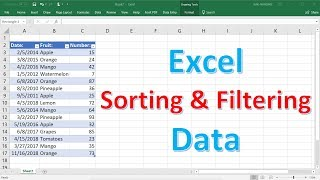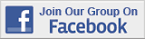Excel Tips & Tutorials:
Descriptive Statistics
 Suggest a Link Alphabetize Page Printer-Friendly List
Watch and Learn
Watch and Learn
Watch and Learn
Watch and Learn
 Sorting and Filtering DataStarting from blank worksheet, from Organic Chemistry Tutor (13:28)How to Use PivotTables to Summarize DataNice introduction and demonstration (17:39).How to Create a Column ChartGood emphasis on design principles (3:40)How to Make a Map ChartDemonstrates using Florida zip codes (4:33).Sorting and Filtering DataStarting from blank worksheet, from Organic Chemistry Tutor (13:28)How to Use PivotTables to Summarize DataNice introduction and demonstration (17:39).How to Create a Column ChartGood emphasis on design principles (3:40)Sorting and Filtering DataStarting from blank worksheet, from Organic Chemistry Tutor (13:28)How to Use PivotTables to Summarize DataNice introduction and demonstration (17:39).Sorting and Filtering DataStarting from blank worksheet, from Organic Chemistry Tutor (13:28)Over 18,000 Members!